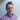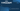Carl Rippon

# Logical Assignment Operators

October 28, 2020
javascripttypescript

We regularly need to implement conditional logic in the apps we build. This post covers a new terse syntax that we can now use for different logical operators in both JavaScript and TypeScript.

## Logical OR assignment

When writing code, we often set a variable to some value if the variable is currently falsy. Consider the example below:

``````function getDefaultValue() {
console.log("getDefaultValue");
return 1;
}

let score: number | null = null;
score = score || getDefaultValue();console.log(score);``````

In this example, `score` is assigned to the result of `getDefaultValue` because `score` was originally falsy.

If `score` is originally truthy, then the second argument is not evaluated:

``````let score: number | null = 9;score = score || getDefaultValue();
console.log(score);``````

The new Logical OR assignment operator gives us a slightly shorter syntax for this pattern:

``````let score: number | null = null;
score ||= getDefaultValue();console.log(score);``````

## Logical nullish assignment

Use of the nullish coalescing operator (`??`) in the previous example means that the default value is only applied if the variable is `null` or `undefined`:

``````let score: number | null = 0;
score = score ?? getDefaultValue();console.log(score);``````

The new Logical nullish assignment operator gives us a slightly shorter syntax for this pattern:

``score ??= getDefaultValue();``

## Logical AND assignment

We regularly use the logical AND operator (`&&`) for expressing conditional logic. Some example code is below:

``````let value = "F";
let valid: boolean = true;
valid = valid && value !== "";valid = valid && value.length > 2;console.log(valid);``````

The new Logical AND assignment operator gives us a slightly shorter syntax for this pattern:

``````valid &&= value !== "";
valid &&= value.length > 2;``````

Nice!

## Wrap up

The new logical assignment operators provide terser syntax for some of the conditional code we write.

The syntax is supported in all major browsers other than IE. Babel supports it with the plugin-proposal-logical-assignment-operators plugin, and TypeScript has recently added support for it in 4.0.

## Did you find this post useful?

Let me know by sharing it on Twitter.